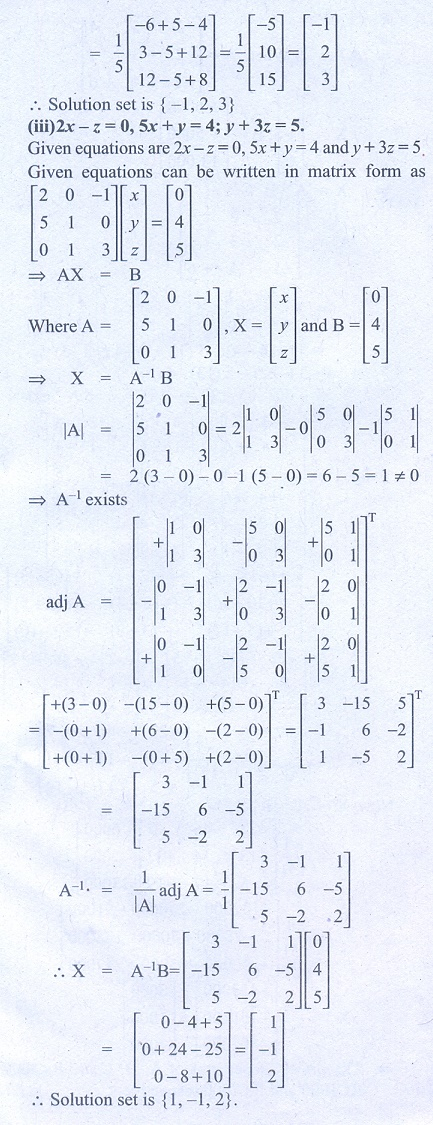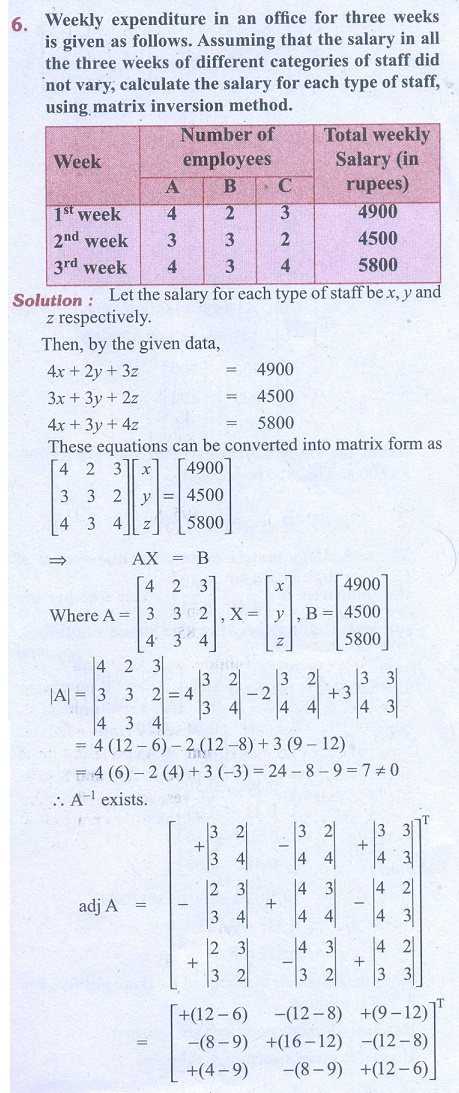Home | | Business Maths 11th std | Exercise 1.3: Solution of a system of linear equations by matrix Inverse Method

# Exercise 1.3: Solution of a system of linear equations by matrix Inverse Method

Business Mathematics and Statistics Book back answers and solution for Exercise questions - Matrices and Determinants: Solution of a system of linear equations by matrix Inverse MethodTags : Problem Questions with Answer, Solution | Business Mathematics , 11th Business Mathematics and Statistics(EMS) : Chapter 1 : Matrices and Determinants
Study Material, Lecturing Notes, Assignment, Reference, Wiki description explanation, brief detail
11th Business Mathematics and Statistics(EMS) : Chapter 1 : Matrices and Determinants : Exercise 1.3: Solution of a system of linear equations by matrix Inverse Method | Problem Questions with Answer, Solution | Business Mathematics